# Decision Tree Algorithm With Example | Decision Tree In Machine Learning | Data Science |Simplilearn

23. Mar 2018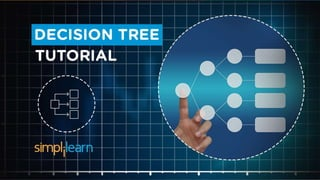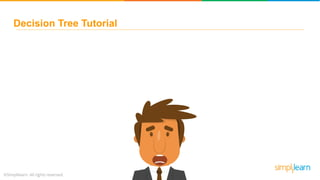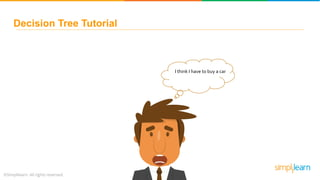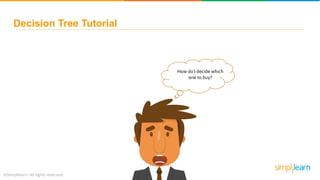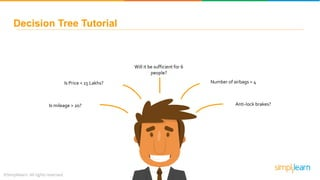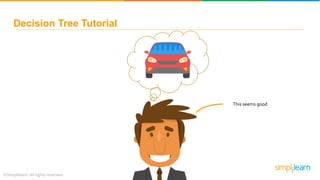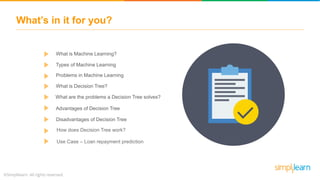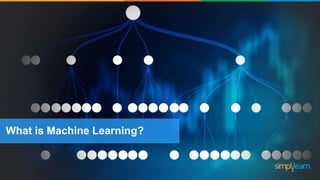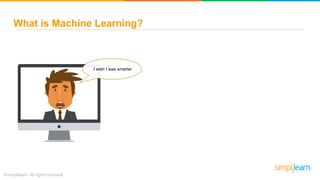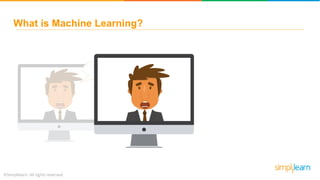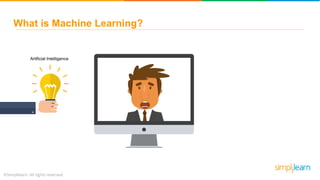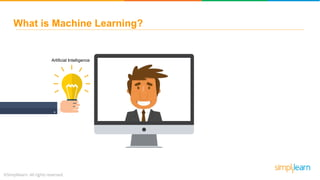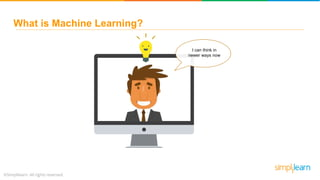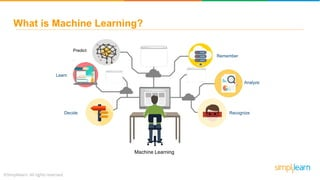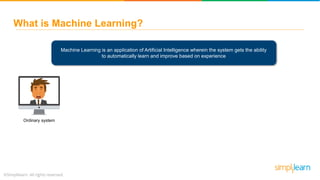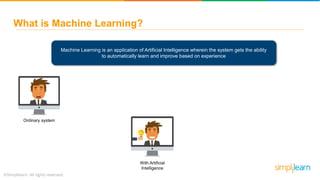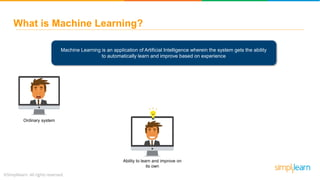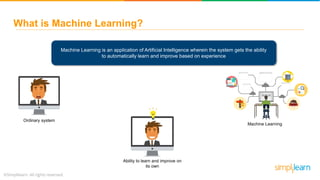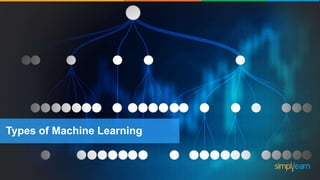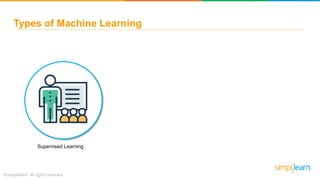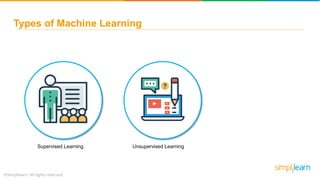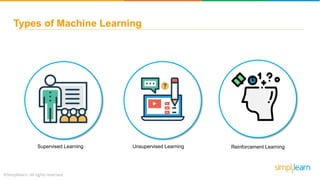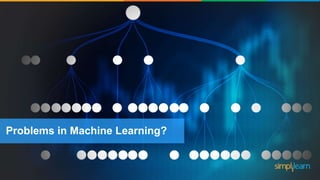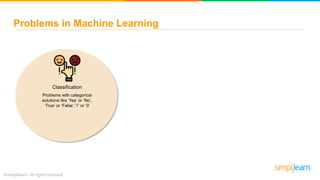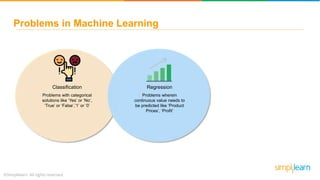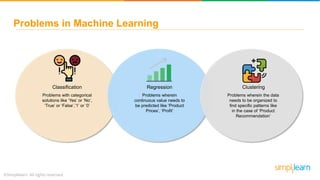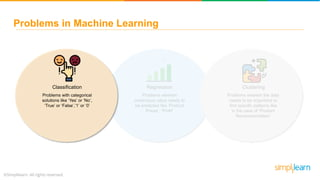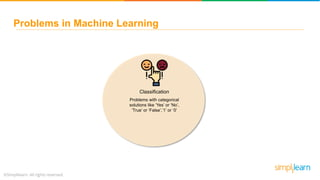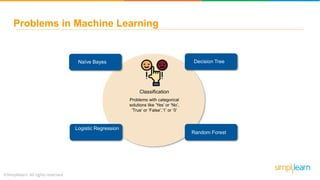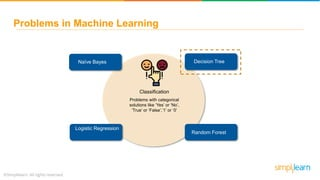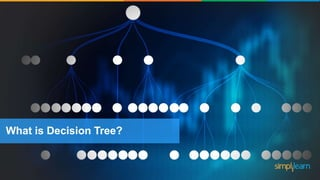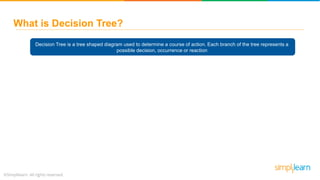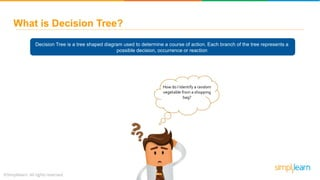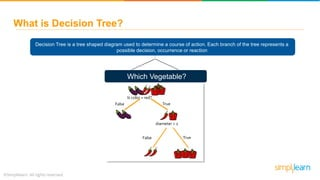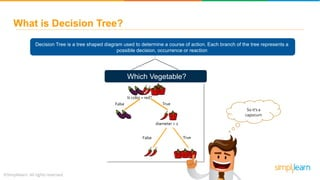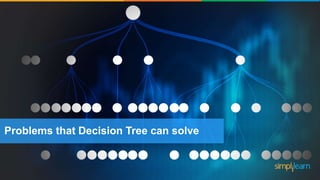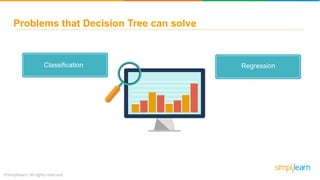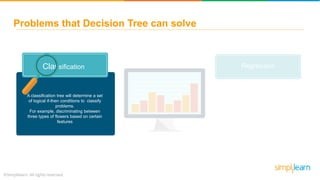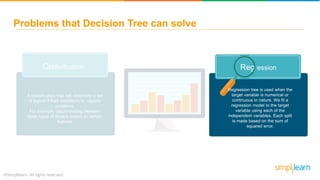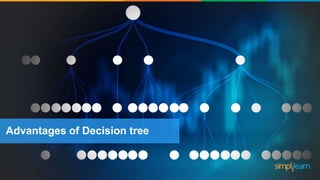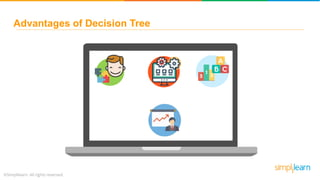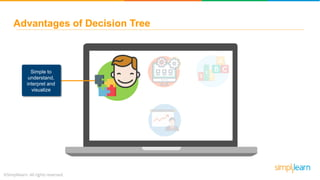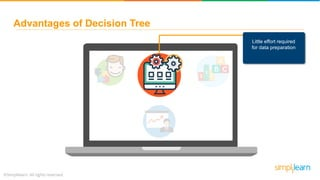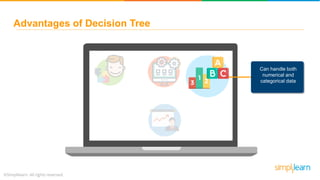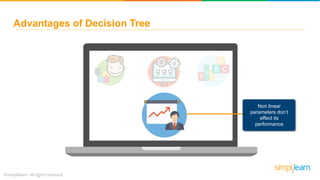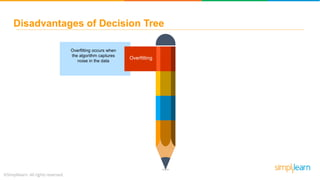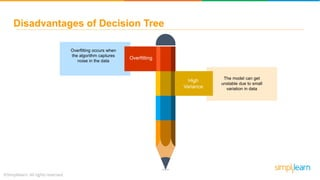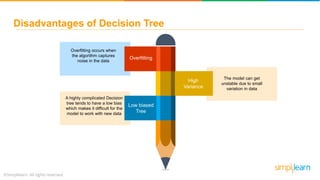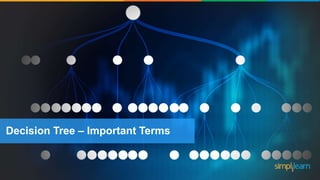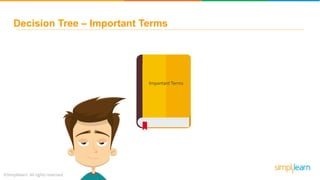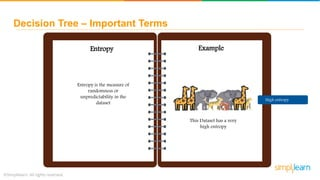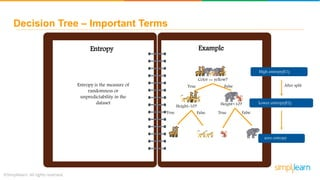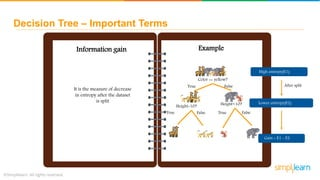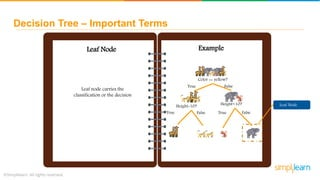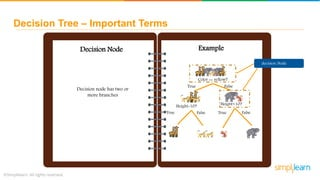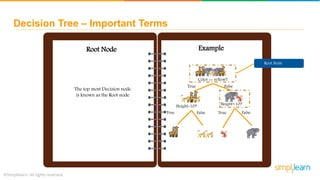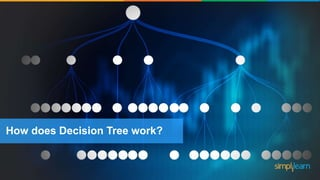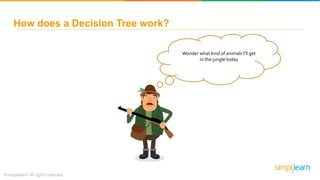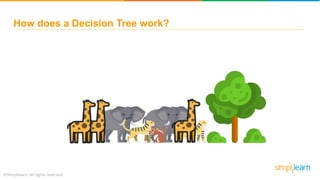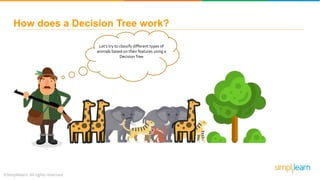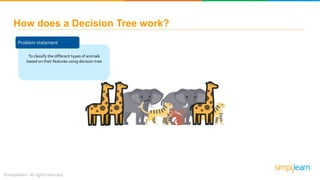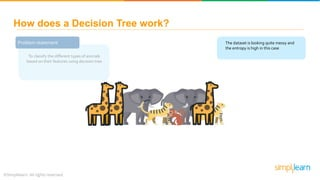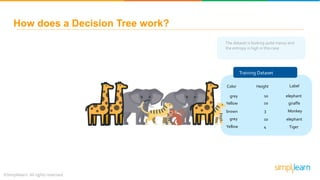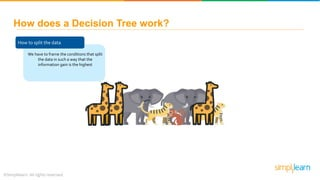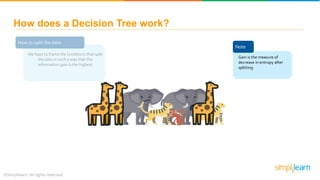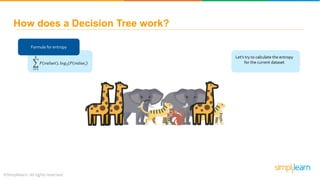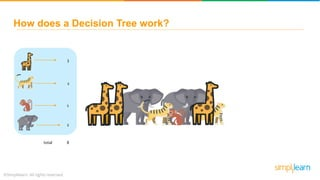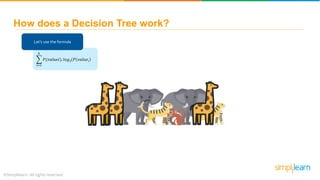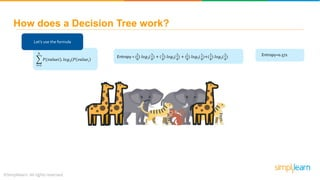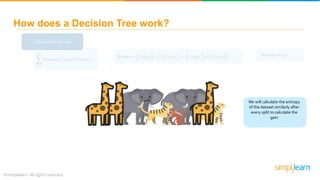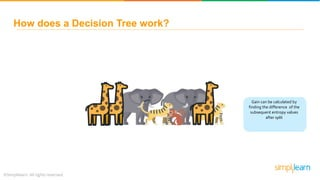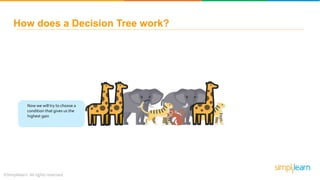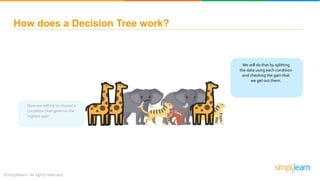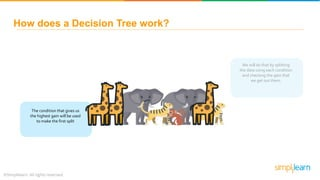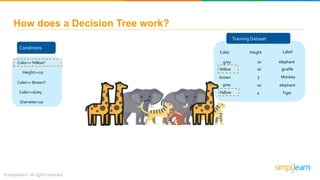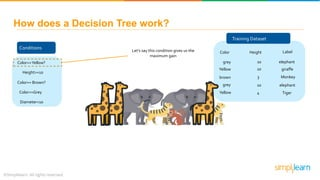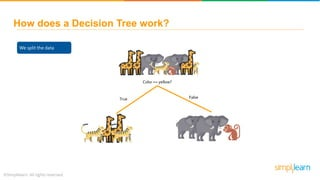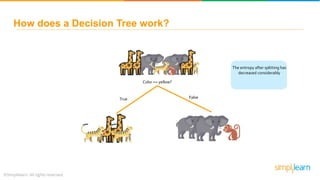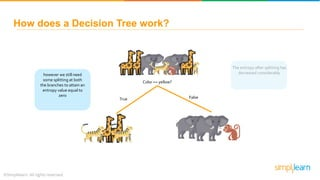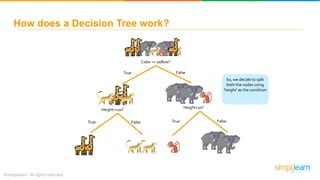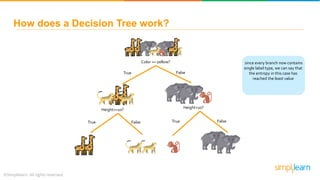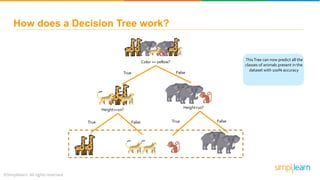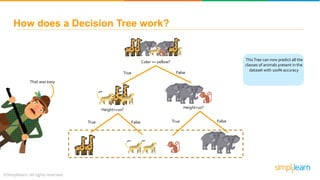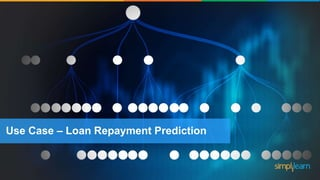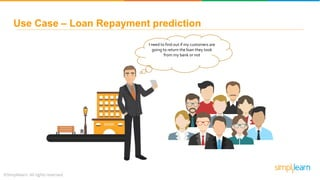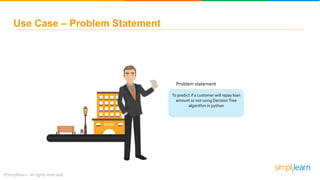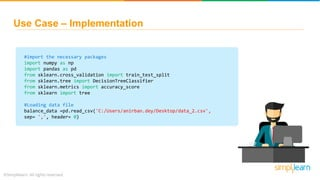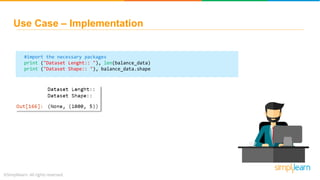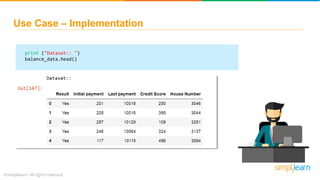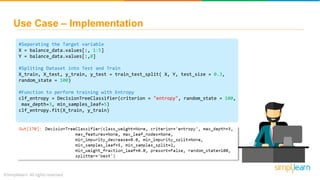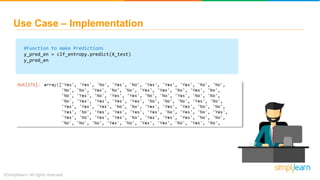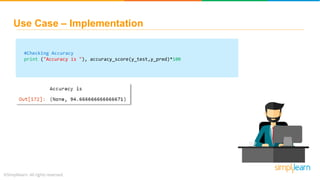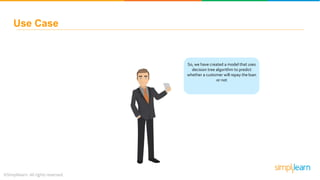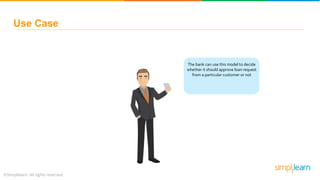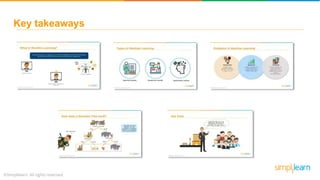1 von 98

### Decision Tree Algorithm With Example | Decision Tree In Machine Learning | Data Science |Simplilearn

• 3. Decision Tree Tutorial I think I have to buy a car
• 4. Decision Tree Tutorial How do I decide which one to buy?
• 5. Decision Tree Tutorial Is mileage > 20? Is Price < 15 Lakhs? Will it be sufficient for 6 people? Number of airbags = 4 Anti-lock brakes?
• 7. What’s in it for you? What is Machine Learning? What is Decision Tree? Problems in Machine Learning What are the problems a Decision Tree solves? Advantages of Decision Tree Disadvantages of Decision Tree How does Decision Tree work? Use Case – Loan repayment prediction Types of Machine Learning
• 8. What is Machine Learning?
• 9. What is Machine Learning? I wish I was smarter
• 10. What is Machine Learning? I wish I was smarter
• 11. What is Machine Learning? Artificial Intelligence
• 12. What is Machine Learning? Artificial Intelligence
• 13. What is Machine Learning? I can think in newer ways now
• 14. What is Machine Learning? Learn Analyze Decide Remember Machine Learning Recognize Predict
• 15. What is Machine Learning? Machine Learning is an application of Artificial Intelligence wherein the system gets the ability to automatically learn and improve based on experience Ordinary system
• 16. What is Machine Learning? Machine Learning is an application of Artificial Intelligence wherein the system gets the ability to automatically learn and improve based on experience Ordinary system With Artificial Intelligence
• 17. What is Machine Learning? Machine Learning is an application of Artificial Intelligence wherein the system gets the ability to automatically learn and improve based on experience Ordinary system Ability to learn and improve on its own
• 18. What is Machine Learning? Machine Learning is an application of Artificial Intelligence wherein the system gets the ability to automatically learn and improve based on experience Ordinary system Ability to learn and improve on its own Machine Learning
• 19. Types of Machine Learning
• 20. Types of Machine Learning Supervised Learning
• 21. Types of Machine Learning Supervised Learning Unsupervised Learning
• 22. Types of Machine Learning Supervised Learning Unsupervised Learning Reinforcement Learning
• 23. Problems in Machine Learning?
• 24. Problems in Machine Learning Classification Problems with categorical solutions like ‘Yes’ or ‘No’, ‘True’ or ‘False’,’1’ or ‘0’
• 25. Problems in Machine Learning Classification Regression Problems with categorical solutions like ‘Yes’ or ‘No’, ‘True’ or ‘False’,’1’ or ‘0’ Problems wherein continuous value needs to be predicted like ‘Product Prices’, ‘Profit’
• 26. Problems in Machine Learning Classification Problems with categorical solutions like ‘Yes’ or ‘No’, ‘True’ or ‘False’,’1’ or ‘0’ Regression Clustering Problems wherein continuous value needs to be predicted like ‘Product Prices’, ‘Profit’ Problems wherein the data needs to be organized to find specific patterns like in the case of ‘Product Recommendation’
• 27. Problems in Machine Learning Regression Clustering Problems wherein continuous value needs to be predicted like ‘Product Prices’, ‘Profit’ Problems wherein the data needs to be organized to find specific patterns like in the case of ‘Product Recommendation’ Classification Problems with categorical solutions like ‘Yes’ or ‘No’, ‘True’ or ‘False’,’1’ or ‘0’
• 28. Problems in Machine Learning Classification Problems with categorical solutions like ‘Yes’ or ‘No’, ‘True’ or ‘False’,’1’ or ‘0’ Decision Tree
• 29. Problems in Machine Learning Classification Problems with categorical solutions like ‘Yes’ or ‘No’, ‘True’ or ‘False’,’1’ or ‘0’ Decision Tree Random Forest Logistic Regression Naïve Bayes
• 30. Problems in Machine Learning Classification Problems with categorical solutions like ‘Yes’ or ‘No’, ‘True’ or ‘False’,’1’ or ‘0’ Decision Tree Random Forest Logistic Regression Naïve Bayes
• 32. What is Decision Tree? Decision Tree is a tree shaped diagram used to determine a course of action. Each branch of the tree represents a possible decision, occurrence or reaction
• 33. What is Decision Tree? Decision Tree is a tree shaped diagram used to determine a course of action. Each branch of the tree represents a possible decision, occurrence or reaction How do I Identify a random vegetable from a shopping bag?
• 34. What is Decision Tree? Decision Tree is a tree shaped diagram used to determine a course of action. Each branch of the tree represents a possible decision, occurrence or reaction Which Vegetable? Is color = red? False True diameter > 2 False True
• 35. What is Decision Tree? Decision Tree is a tree shaped diagram used to determine a course of action. Each branch of the tree represents a possible decision, occurrence or reaction Which Vegetable? So it’s a capsicum Is color = red? False True diameter > 2 False True
• 36. Problems that Decision Tree can solve
• 37. Problems that Decision Tree can solve RegressionClassification
• 38. Problems that Decision Tree can solve RegressionClassification A classification tree will determine a set of logical if-then conditions to classify problems. For example, discriminating between three types of flowers based on certain features
• 39. Problems that Decision Tree can solve RegressionClassification Regression tree is used when the target variable is numerical or continuous in nature. We fit a regression model to the target variable using each of the independent variables. Each split is made based on the sum of squared error. A classification tree will determine a set of logical if-then conditions to classify problems. For example, discriminating between three types of flowers based on certain features
• 42. Advantages of Decision Tree Simple to understand, interpret and visualize
• 43. Advantages of Decision Tree Little effort required for data preparation
• 44. Advantages of Decision Tree Can handle both numerical and categorical data
• 45. Advantages of Decision Tree Non linear parameters don’t effect its performance
• 47. Disadvantages of Decision Tree Overfitting Map Overfitting occurs when the algorithm captures noise in the data
• 48. Overfitting occurs when the algorithm captures noise in the data Disadvantages of Decision Tree Overfitting High Variance Map The model can get unstable due to small variation in data
• 49. Overfitting occurs when the algorithm captures noise in the data The model can get unstable due to small variation in data Disadvantages of Decision Tree Overfitting High Variance Low biased Tree Map A highly complicated Decision tree tends to have a low bias which makes it difficult for the model to work with new data
• 50. Decision Tree – Important Terms
• 51. Decision Tree – Important Terms ImportantTerms
• 52. Decision Tree – Important Terms Entropy Example Entropy is the measure of randomness or unpredictability in the dataset This Dataset has a very high entropy High entropy
• 53. Decision Tree – Important Terms Entropy Example Entropy is the measure of randomness or unpredictability in the dataset High entropy(E1) Color == yellow? True False Height=10? Height<10? True TrueFalse False Lower entropy(E2) zero entropy After split
• 54. Decision Tree – Important Terms Information gain Example High entropy(E1) Color == yellow? True False Height=10? Height<10? True TrueFalse False Lower entropy(E2) Gain = E1 - E2 After split It is the measure of decrease in entropy after the dataset is split
• 55. Decision Tree – Important Terms Leaf Node Example Leaf Node Color == yellow? True False Height=10? Height<10? True TrueFalse False Leaf node carries the classification or the decision
• 56. Decision Tree – Important Terms Decision Node Example decision Node Color == yellow? True False Height=10? Height<10? True TrueFalse False Decision node has two or more branches
• 57. Decision Tree – Important Terms Root Node Example Root Node Color == yellow? True False Height=10? Height<10? True TrueFalse False The top most Decision node is known as the Root node
• 58. How does Decision Tree work?
• 59. Wonder what kind of animals I’ll get in the jungle today How does a Decision Tree work?
• 60. How does a Decision Tree work?
• 61. How does a Decision Tree work? Let’s try to classify different types of animals based on their features using a DecisionTree
• 62. Problem statement To classify the different types of animals based on their features using decision tree How does a Decision Tree work?
• 63. The dataset is looking quite messy and the entropy is high in this case How does a Decision Tree work? Problem statement To classify the different types of animals based on their features using decision tree
• 64. The dataset is looking quite messy and the entropy is high in this case Training Dataset Color Height Label grey Yellow brown grey Yellow 10 3 10 10 4 elephant elephant giraffe Tiger Monkey How does a Decision Tree work?
• 65. How to split the data We have to frame the conditions that split the data in such a way that the information gain is the highest How does a Decision Tree work?
• 66. How to split the data We have to frame the conditions that split the data in such a way that the information gain is the highest Note Gain is the measure of decrease in entropy after splitting How does a Decision Tree work?
• 67. 𝑖=1 𝑘 𝑃(𝑣𝑎𝑙𝑢𝑒𝑖). 𝑙𝑜𝑔2(𝑃 𝑣𝑎𝑙𝑢𝑒𝑖 How does a Decision Tree work? Formula for entropy Let’s try to calculate the entropy for the current dataset
• 68. How does a Decision Tree work? total 3 2 1 2 8
• 69. How does a Decision Tree work? 𝑖=1 𝑘 𝑃(𝑣𝑎𝑙𝑢𝑒𝑖). 𝑙𝑜𝑔2(𝑃 𝑣𝑎𝑙𝑢𝑒𝑖 Let’s use the formula
• 70. How does a Decision Tree work? 𝑖=1 𝑘 𝑃(𝑣𝑎𝑙𝑢𝑒𝑖). 𝑙𝑜𝑔2(𝑃 𝑣𝑎𝑙𝑢𝑒𝑖 Let’s use the formula Entropy = ( 3 8 ) 𝑙𝑜𝑔2( 3 8 ) + ( 2 8 ) 𝑙𝑜𝑔2( 2 8 ) + ( 1 8 ) 𝑙𝑜𝑔2( 1 8 )+( 2 8 ) 𝑙𝑜𝑔2( 2 8 ) Entropy=0.571
• 71. How does a Decision Tree work? 𝑖=1 𝑘 𝑃(𝑣𝑎𝑙𝑢𝑒𝑖). 𝑙𝑜𝑔2(𝑃 𝑣𝑎𝑙𝑢𝑒𝑖 Let’s use the formula Entropy = ( 3 8 ) 𝑙𝑜𝑔2( 3 8 ) + ( 2 8 ) 𝑙𝑜𝑔2( 2 8 ) + ( 1 8 ) 𝑙𝑜𝑔2( 1 8 )+( 2 8 ) 𝑙𝑜𝑔2( 2 8 ) Entropy=0.571 We will calculate the entropy of the dataset similarly after every split to calculate the gain
• 72. How does a Decision Tree work? Gain can be calculated by finding the difference of the subsequent entropy values after split
• 73. Now we will try to choose a condition that gives us the highest gain How does a Decision Tree work?
• 74. Now we will try to choose a condition that gives us the highest gain We will do that by splitting the data using each condition and checking the gain that we get out them. How does a Decision Tree work?
• 75. We will do that by splitting the data using each condition and checking the gain that we get out them. The condition that gives us the highest gain will be used to make the first split How does a Decision Tree work?
• 76. Training Dataset Color Height Label grey Yellow brown grey Yellow 10 3 10 10 4 elephant elephant giraffe Tiger Monkey How does a Decision Tree work? Conditions Color==Yellow? Height>=10 Color== Brown? Color==Grey Diameter<10
• 77. Let’s say this condition gives us the maximum gain How does a Decision Tree work? Conditions Color==Yellow? Height>=10 Color== Brown? Color==Grey Diameter<10 Training Dataset Color Height Label grey Yellow brown grey Yellow 10 3 10 10 4 elephant elephant giraffe Tiger Monkey
• 78. We split the data How does a Decision Tree work? Color == yellow? True False
• 79. The entropy after splitting has decreased considerably How does a Decision Tree work? Color == yellow? True False
• 80. The entropy after splitting has decreased considerablyhowever we still need some splitting at both the branches to attain an entropy value equal to zero How does a Decision Tree work? Color == yellow? True False
• 81. So, we decide to split both the nodes using ‘height’ as the condition How does a Decision Tree work? Color == yellow? True False Height>=10? Height<10? True TrueFalse False
• 82. since every branch now contains single label type, we can say that the entropy in this case has reached the least value How does a Decision Tree work? Color == yellow? True False Height>=10? Height<10? True TrueFalse False
• 83. How does a Decision Tree work? Color == yellow? True False Height>=10? Height<10? True TrueFalse False ThisTree can now predict all the classes of animals present in the dataset with 100% accuracy
• 84. How does a Decision Tree work? Color == yellow? True False Height>=10? Height<10? True TrueFalse False ThisTree can now predict all the classes of animals present in the dataset with 100% accuracy That was easy
• 85. Use Case – Loan Repayment Prediction
• 86. Use Case – Loan Repayment prediction I need to find out if my customers are going to return the loan they took from my bank or not
• 87. Use Case – Problem Statement Problem statement To predict if a customer will repay loan amount or not using DecisionTree algorithm in python
• 88. Use Case – Implementation #import the necessary packages import numpy as np import pandas as pd from sklearn.cross_validation import train_test_split from sklearn.tree import DecisionTreeClassifier from sklearn.metrics import accuracy_score from sklearn import tree #Loading data file balance_data =pd.read_csv('C:/Users/anirban.dey/Desktop/data_2.csv', sep= ',', header= 0)
• 89. Use Case – Implementation #import the necessary packages print ("Dataset Lenght:: "), len(balance_data) print ("Dataset Shape:: "), balance_data.shape
• 90. Use Case – Implementation print ("Dataset:: ") balance_data.head()
• 91. Use Case – Implementation #Seperating the Target variable X = balance_data.values[:, 1:5] Y = balance_data.values[:,0] #Spliting Dataset into Test and Train X_train, X_test, y_train, y_test = train_test_split( X, Y, test_size = 0.3, random_state = 100) #Function to perform training with Entropy clf_entropy = DecisionTreeClassifier(criterion = "entropy", random_state = 100, max_depth=3, min_samples_leaf=5) clf_entropy.fit(X_train, y_train)
• 92. Use Case – Implementation #Function to make Predictions y_pred_en = clf_entropy.predict(X_test) y_pred_en
• 93. Use Case – Implementation #Checking Accuracy print ("Accuracy is "), accuracy_score(y_test,y_pred)*100
• 94. Use Case So, we have created a model that uses decision tree algorithm to predict whether a customer will repay the loan or not
• 95. Use Case The Accuracy of the model is 94.6%
• 96. Use Case The bank can use this model to decide whether it should approve loan request from a particular customer or not

1. Style - 01
2. Style - 01
3. Style - 01
4. Style - 01
5. Style - 01
6. Style - 01
7. Style - 01
8. Style - 01
9. Style - 01
10. Style - 01
11. Style - 01
12. Style - 01
13. Style - 01
14. Style - 01
15. Style - 01
16. Style - 01
17. Style - 01
18. Style - 01
19. Style - 01
20. Style - 01
21. Style - 01
22. Style - 01
23. Style - 01
24. Style - 01
25. Style - 01
26. Style - 01
27. Style - 01
28. Style - 01
29. Style - 01
30. Style - 01
31. Style - 01
32. Style - 01
33. Style - 01
34. Style - 01
35. Style - 01
36. Style - 01
37. Style - 01
38. Style - 01
39. Style - 01
40. Style - 01
41. Style - 01
42. Style - 01
43. Style - 01
44. Style - 01
45. Style - 01
46. Style - 01
47. Style - 01
48. Style - 01
49. Style - 01
50. Style - 01
51. Style - 01
52. Style - 01
53. Style - 01
54. Style - 01
55. Style - 01
56. Style - 01
57. Style - 01
58. Style - 01
59. Style - 01
60. Style - 01
61. Style - 01
62. Style - 01
63. Style - 01
64. Style - 01
65. Style - 01
66. Style - 01
67. Style - 01
68. Style - 01
69. Style - 01
70. Style - 01
71. Style - 01
72. Style - 01
73. Style - 01
74. Style - 01
75. Style - 01
76. Style - 01
77. Style - 01
78. Style - 01
79. Style - 01
80. Style - 01
81. Style - 01
82. Style - 01
83. Style - 01
84. Style - 01
85. Style - 01
86. Style - 01